or# RD Sharma Class 9 and Chapter Rationalisation Maths Solutions

## Maths Class 9 and Chapter Rationalisation RD Sharma SolutionsFilter
Filters :
CLASSES
•
•
•
•
•
•
•
CHAPTERS
•
• 15 More

### RD SHARMA Class 9 | RATIONALISATION

Rationales the denominator and simplify: `(sqrt(3)-sqrt(2))/(sqrt(3)+sqrt(2))` (ii) `(5+2sqrt(3))/(7+4sqrt(3))` (iii) `(1+sqrt(2))/(3-2sqrt(2))` `(2sqrt(6)-sqrt(5))/(3sqrt(5)-2sqrt(6))` (v) `(4sqrt(3)+5sqrt(2))/(sqrt(48)+sqrt(18))` (vi) `(2sqrt(3)-sqrt(5))/(2sqrt(3)+3sqrt(3))`# RD Sharma Class 9 Solutions Chapter 3 Rationalisation

In class 7 Maths, through the previous units, the concepts like the number system, real numbers, exponents were covered in-depth in the RD Sharma’s book. By now the students must have got a sound knowledge about the rational and irrational numbers, too. This chapter covers one of the important operations that involve the irrational and rational numbers. The rationalisation is a concept where the denominators of a fractional number are converted into a rational number. All the vital concepts in this chapter are well explained in RD Sharma’s books along with plenty of examples and exercises.

RD Sharma’s books have been one of the most preferred textbooks in India by the students studying the CBSE syllabus. The students throughout find it really easy to understand when learnt from RD Sharma’s book, especially a subject like Maths. This is owing to the reason that RD Sharma’s books provide an easy explanation to even the difficult concepts. The step-by-step approach followed to solve the problems is the highlight of the textbook and it makes the process of self-study tad easy for the learners.

RD Sharma’s books cover all that required to face not just the CBSE academic exams, but also crack the other competitive entrance examinations such as the IIT-JEE, BITSAT, VITEEE, etc. Class 9 marks the beginning of a crucial period that requires serious preparations from the students who aspire to crack these tough entrance exams and RD Sharma’s books serve as the guiding light for them.

For a subject like Maths, it is essential that the learners solve more and more problems in order to learn any chapter better. The RD Sharma’s textbook provides exercise problems that are sufficient for the students’ practise. Now with a proper textbook for reference, the next important requirement for the students would be a good solution guide to the exercise questions from the textbook. The solutions guide proves to be very useful when the students try to solve the difficult textbook questions. With the help of a proper solutions guide, the students who are into self-study would benefit a lot.

Our online tutorial platform Doubtnut provides all the solutions worked out for the RD Sharma textbook, exercise-wise. RD Sharma Class 9 Solutions Chapter 3 Rationalisation on doubtnut.com are presented in the form of videos that can be accessed from anywhere and at any time. The students can bank on these solution videos as all problems, including the complex ones are broken down into simpler solutions.

## Rationalisation – Class 9 Maths RD Sharma Solutions

The word ‘Rationalisation’ itself indicates that there is something to do with rational and irrational numbers. Rational and irrational numbers form an important part of the number system. So, before we get into the chapter of Rationalisation, let us recollect the terms rational and irrational numbers.

### What are the rational numbers?

Rational numbers are the ones that can be expressed of the form p/q, where ‘p’ and ‘q’ are integers. For example, 1, 2/3, -5/4, 2, 3, etc, are the rational numbers.

### What are the irrational numbers?

Irrational numbers are the ones that cannot be expressed in the form of p/q where ‘p’ and ‘q’ are integers, or as any integers. The irrational numbers don’t end up being periodic or terminate.  Example, the value of Pi that is expressed as 3.14, which is a rounded off decimal, which otherwise doesn’t terminate and hence this forms an irrational number. Another example of an irrational number is the square root of two that actually goes on to infinity and is rounded off to a value that approximately equals to 1.414. And this also reveals that most of the square roots of the natural numbers are irrational.

Moving on to the problems and operations that involve the real numbers, there are high chances that the students would have to deal with both rational and irrational numbers. Solving the problems that involve irrational numbers might seem a little tricky, but with proper guidance from platforms like the Doubtnut, such complex concepts can be mastered in no time.

### What actually is rationalisation?

Talking of the problems consisting of the irrational numbers, it is quite tough to arrive at solutions, especially when the irrational numbers take the position of denominators. So, when a number has an irrational denominator, things become a little complex and operations using such numbers become a little more difficult to solve. It is always advisable to convert such irrational denominators into easily solvable forms. Hence the number that has an irrational denominator has to be rationalised in order to solve the problems in simple ways. This way, a fractional number with an irrational can be easily rewritten into a number containing a rational denominator through this process of rationalisation.

Let’s take an example of the number, 1/√2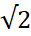. This number can be rationalised by multiplying the numerator and the denominator by √2. This gives, (1x√2)/( √2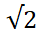√2) = √2/2. And now, the denominator can be said to be rationalised. This gives the simplest form of expressing the number.

All the problems in this unit are based on this concept of rationalising the denominators. And there arise a few situations when certain relevant formulae and rules pertaining to rationalisation would have to be applied.

Basically, rationalising a fraction has more to do with if the denominator is monomial or binomial. A monomial denominator is simpler to rationalise than a binomial denominator.

#### Monomial denominators

Monomial denominators would consist of simply one irrational number and nothing more.

Now, take a/√b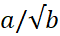. Here,  √b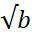is a monomial denominator which can be easily rationalised by multiplied by b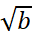on both numerator and denominator. a/√b= ab* bb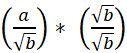= ab/b.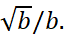Thus rationalising the denominator.

#### Binomial denominators

Binomial denominators would consist of two terms that include an irrational number. The rationalisation would involve multiplying the numerator and denominator by taking the conjugate.

Taking an example of a number consisting of binomial denominator,

1 / (a+b)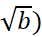= 1a+b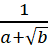x  a -ba-b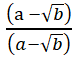= (a - b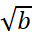) / (a2 – b). Thus, reducing the irrational denominator into a rational number. Here, (a - b) forms the conjugate the irrational denominator (a + b).

In order to rationalise, there would often arise the need to make use of certain algebraic formulae such as (a + b) (a - b) = a2 – b2.

This chapter covers various examples and exercises that require the students to learn the process of taking conjugate and solving the problems. A lot of practise is required in handling problems with complex binomial denominators. The exercise problems in RD Sharma’s are more than sufficient in learning Rationalisation.

And in further classes, this chapter forms as a base in solving complex equations by takin conjugates, applying the relevant formulae, etc. One can expect a lot of questions from this unit on the competitive entrance exams, too. The students can take help from doubtnut.com to arrive at the solutions for the RD Sharma book problems that are solved exercise-wise.

## Topics and Subtopics of RD Sharma Class 9 Maths Chapter 3 – Rationalisation

The rationalisation is more of a technique-based chapter and there are very few topics and subtopics to go about. There are hardly one or two techniques in this chapter that are important to know and the whole unit is about using these few topics according to the questions asked. Knowing the topics and subtopics of a given chapter helps the learners be aware of the whole content in a nutshell. This helps them to sort out each topic the way they want to while planning to study. Let us look at the topics and Subtopics of RD Sharma Class 9 Maths Chapter 3 – Rationalisation, according to which the solutions are worked out on the doubtnut.com:

### Application of extended laws of exponents to real numbers

This topic covers all the simple problems that involve using the certain laws of exponents and formulae such as (a + b) (a - b) = a2 – b2 and (a + b)2 = (a2+2×a×b+b2), in order to arrive at the solutions.

### Rationalisation of real numbers

Problems involving the rationalisation of the denominators can be found here. The problems found here include using both the simple concepts as well as the concept that includes the multiplication of conjugates.

As it can be seen, it might sound difficult in the beginning but with the help of right study medium and materials, the students can easily master even the complex concepts. On doubtnut.com, all of these concepts are taught in video format and this helps the students to learn the lessons with better concentration. The lessons taught in a step-by-step manner by an expert teacher helps in better retention, too. All the doubts occurring during the learning process can be easily clarified by just posting the picture of the question on the Doubtnut. Thus, this is the right platform especially for the students who are into self-study. Doubtnut makes the perfect tutorial platform for the students to face both board exams and the competitive exams in a tension-free way!